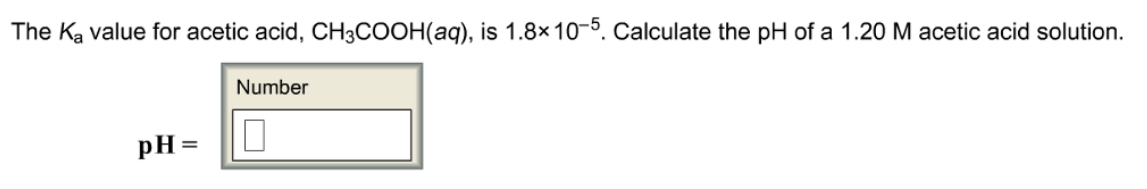# Problem: The Ka value for acetic acid, CH3COOH(aq), is 1.8 x 10-5. Calculate the pH of a 1.20 M acetic acid solution. Calculate the pH of the resulting solution when 3.50 mL of the 1.20 M acetic acid is diluted to make a 250.0 mL solution.

###### FREE Expert Solution
92% (222 ratings)###### Problem Details

The Ka value for acetic acid, CH3COOH(aq), is 1.8 x 10-5. Calculate the pH of a 1.20 M acetic acid solution.

Calculate the pH of the resulting solution when 3.50 mL of the 1.20 M acetic acid is diluted to make a 250.0 mL solution.